Download Presentation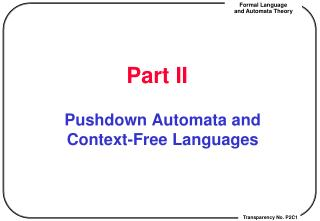Part II

# Part II - PowerPoint PPT Presentation

Part II. Pushdown Automata and Context-Free Languages. Chapter 1. Context-Free Grammars and Languages (Lecture 19,20). Bacus-Naur Form. Regular Expr is too limited to describe practical programming languages. Bacus-Naur Form (BNF)I am the owner, or an agent authorized to act on behalf of the owner, of the copyrighted work described.
Download Presentation## Part II

An Image/Link below is provided (as is) to download presentation

Download Policy: Content on the Website is provided to you AS IS for your information and personal use and may not be sold / licensed / shared on other websites without getting consent from its author.While downloading, if for some reason you are not able to download a presentation, the publisher may have deleted the file from their server.

- - - - - - - - - - - - - - - - - - - - - - - - - - E N D - - - - - - - - - - - - - - - - - - - - - - - - - -
Presentation Transcript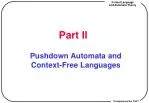### Part II

Pushdown Automata and Context-Free Languages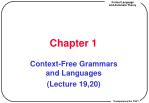### Chapter 1

Context-Free Grammars and Languages

(Lecture 19,20)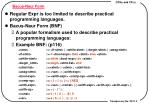Bacus-Naur Form
• Regular Expr is too limited to describe practical programming languages.
• Bacus-Naur Form (BNF)
• A popular formalism used to describe practical programming languages:
• Example BNF: (p116)

<stmt> ::= <if-stmt> | <while-stmt> | <begin-stmt> | <assg-stmt>

<if-stmt> ::= if <bool-expr> then <stmt> else <stmt>

<while-stmt> ::= while <bool-expr> do <stmt>

<begin_stmt> ::= begin <stmt-list> end

<stmt-list> ::=<stmt> | <stmt> ; <stmt-list>

<assg-stmt> ::= <var> := <arith-expr>

<bool-expr> ::= <arith-expr> <comp-op> <arith-expr>

<comp-op> ::= < | > | <= | >= | NEQ

<arith-expr> ::= <var> | <const> | ( <arith-expr> <arith-op> <arith-expr> )

<arith-op> ::= + | - | * | /

<const> ::= 0 | 1 | 2 | ... |8 | 9

<var> ::= a | b | c |... |x | y | z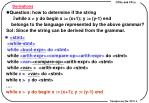Derivations
• Question: how to determine if the string
• while x > y do begin x := (x+1); y := (y-1) end

belongs to the language represented by the above grammar?

Sol: Since the string can be derived from the grammar.

• <stmt>

<while-stmt>

while <bool-expr> do <stmt>

while <arith-expr><compare-op><arith-expr> do <stmt>

while <var><compare-op><arith-expr> do <stmt>

while <var> > <arith-expr> do <stmt>

while x > <var> do <stmt>

while x > y do <stmt>

….

while x > y do begin x := (x+1); y := (y-1) end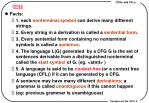CFGs
• Facts:
• 1. each nonterminal symbol can derive many different strings.
• 2. Every string in a derivation is called a sentential form.
• 3. Every sentential form containing no nonterminal symbols is called a sentence.
• 4. The language L(G) generated by a CFG G is the set of sentences derivable from a distinguished nonterminal called the start symbol of G. (eg. <stmt> )
• 5. A language is said to be context free (or a context free language (CFL)) if it can be generated by a CFG.
• A sentence may have many different derivations; a grammar is called unambiguous if this cannot happen
• (eg: previous grammar is unambiguous)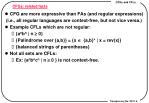CFGs: related facts
• CFG are more expressive than FAs (and regular expressions)

(i.e., all regular languages are context-free, but not vice versa.)

• Example CFLs which are not regular:
• {anbn | n  0}
• {Palindrome over {a,b}} = {x {a,b}* | x = rev(x)}
• {balanced strings of parentheses}
• Not all sets are CFLs:
• Ex: {anbncn | n  0 } is not context-free.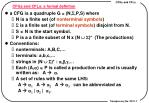CFGs and CFLs: a formal defintion
• a CFG is a quadruple G = (N,S,P,S) where
• N is a finite set (of nonterminal symbols)
• S is a finite set (of terminal symbols) disjoint from N.
• S  N is the start symbol.
• P is a a finite subset of N x (N S)* (The productions)
• Conventions:
• nonterminals: A,B,C,…
• terminals: a,b,c,…
• strings in (N S)* : a,b,g,…
• Each (A,a)  P is called a production rule and is usually written as: A a.
• A set of rules with the same LHS:

A a1 A a2 A a3 can be abbreviated as

A a1| a2 | a3.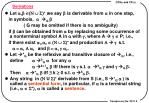Derivations
• Let a,b (N  S)* we say b is derivable from a in one step,

in symbols, a G b

( G may be omitted if there is no ambiguity)

if b can be obtained from a by replacing some occurrence of a nonterminal symbol A in a with g, where A g P; i.e.,

if there exist a1,a2 (N  S)*and production A  g s.t.

a = a1 A a2and b = a1 g a2.

• Let *G be the reflexive and transitive closure of G, i.e.,

define a0Ga for any a

ak+1Gb iff there is g s.t. akGg and gG b.

Then a*Gb iff \$ k  0 s.t. akGb.

• Any string in (N U S)*derivable from S (i.e., S*G a ) is called a sentential form, in particular, if a is a terminal string (i.e., a  S*), a is called a sentence.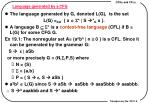Language generated by a CFG
• The language generated by G, denoted L(G), is the set

L(G) =def { x S* | S *G x }.

• A language B S* is a context-free language (CFL) if B = L(G) for some CFG G.

Ex 19.1: The nonregular set A= {anbn | n  0 } is a CFL. Since it can be generated by the grammar G:

S e | aSb

or more precisely G = (N,S,P,S) where

• N = {S}
• S = {a,b}
• P = { S e, S  aSb }
• a3b3 L(G) since S  aSb  aaSbb  aaaSbbb  aaabbb.

 S 4 aaabbb and S * aaabbb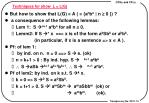Techniques for show L = L(G)
• But how to show that L(G) = A ( = {anbn | n  0 }) ?
• a consequence of the following lemmas:
• Lem 1: S n+1 anbn for all n  0.
• Lemm2: If S * x ==> x is of the form akSbk or akbk.

(in particular, if x is a sentence => x  A ).

• Pf: of lem 1:
• by ind. on n. n = 0 ==> S  e. (ok)
• n = k+1 > 0 : By ind. hyp. S k+1 akbk
•  S aSb k+1 ak+1bk+1.  S n+1 anbn.
• Pf of lem2: by ind. on k s.t. S k x.
• k = 0 => S 0 S = a0Sb0.
• k = t+1 > 0. S t amSbm amaSbbm (ok) or
•  ambm. (ok).Balanced Parentheses

Ex 19.2: The set of palindromes P = { x  {a,b}* | x = rev(x) }.

can be generated by the grammar G:

S e | a | b | aSa | bSb.

cf: The inductive definition of P

1. Initial condition: e, a and b are palindromes.

2. recursive condition:

If S is a palindrome, then so are aSa and bSb.

• Balanced Parentheses:

Ex1: 2+3x5-4x6 ==> ((2+3)x(5-(4x6)))

==> (( ) ( ( )))

--- balanced parentheses.

Ex2: unbalanced parentheses:

( ( )) ((( )) ))) --- no of “(“  no of “)”.

( ( ) ( ( ) ) )) ((( )) --- unmatched “)” encountered.Balanced Parentheses
• Formal definition:
• let S { [, ] }. Define L,R: S*  N as follows:
• L(x) = number of “[“ in x.
• R(x) = number of “]” in x.
• a string x S* is said to be balanced iff

(i) L(x) = R(x) -- equal # of left and right parentheses.

(ii) for all prefix y of x, L(y)  R(y).

--- no dangling right parenthesis.

• Now define PAREN = { x  { [, ] }* | x is balanced }.
• Thm 20.1 : PAREN can be generated by the CFG G:

S e | [S] | S S

pf: 1. L(G)  PAREN.

Lem1: If S  * x then x is balanced. In particular, if x contains no S => x  PAREN.  L(G)  PAREN.proof of theorem 20.1

pf of lem1 : by ind. on k s.t. S k x.

k = 0 => S 0 S = x is balanced.

k = t + 1 > 0:

==> S t ySz  yz (1) or

 y[S]z (2) or

 ySSz (3).

By ind. hyp., ySz is balanced.

=> L(yz) = L(ySz) = R(ySz) = R(yz) and

if y = wu => L(w)  R(w) since w is also a prefix of ySz.

if z = wu => L(yw) = L(ySw)  R(ySw) = R(yw).

 yz is balanced.

Case (2) and (3) can be proved similarly.Proof of theoreom 20.1 (cont’d)

Pf: PAREN  L(G) (i.e., if x is balanced ==> S  * x. )

By ind. on |x|.

1. |x| = 0 ==> x = e ==> S e (ok).

2. |x| > 0. Then either

(a) \$ a proper prefix y of x that is balanced or

(b) No proper prefixes y of x are balanced.

• In case (a), we have x = y z with |y|,|z| < |x| for some z.

=> L(z) = L(x) - L(y) = R(x) - R(y) = R(z)

For all prefix w with z = w w’: L(w) = L(yw) - L(y)  R(yw) - R(y) = R(w)

==> both y and z are balanced ==> by ind. hyp., S * y and S * z

==> S  SS * yS *yz.

In case (b): x = [z] for some z (why ?)

Moreover it can be shown that z is balanced too.

Hence S * z. ==> S * [S] * [z] = x. QEDPushdown Automata: a preview
• FAs recognize regular languages.
• What kinds of machines recognize CFLs ?

===> Pushdown automata (PDAs)

• PDA:
• Like FAs but with an additionalstack as working memory.
• Actions of a PDA

1. Move right one tape cell (as usual FAs)

2. push a symbol onto stack

3. pop a symbol from the stack.

• Actions of a PDA depend on

1. current state 2. currently scanned I/P symbol

3. current top stack symbol.

• A string x is accepted by a PDA if it can enter a final state (or clear all stack symbols) after scanning the entire input.
• More details defer to later chapters.